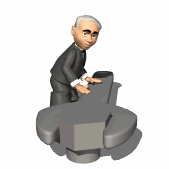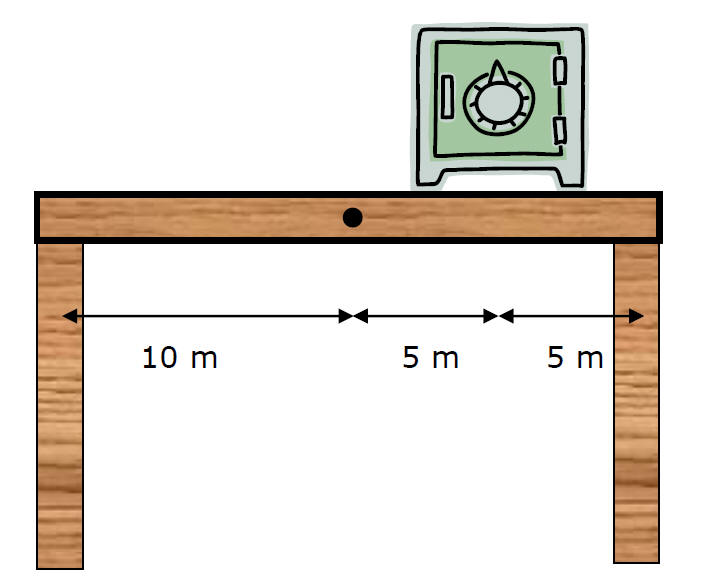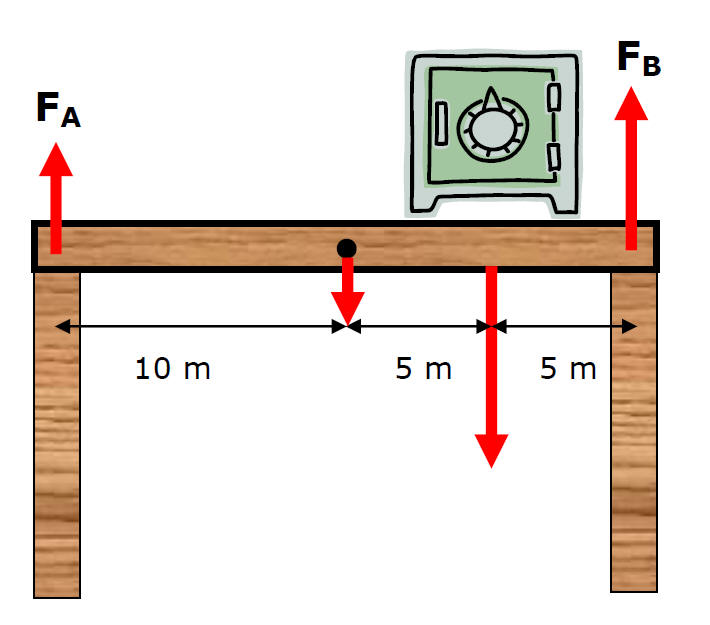HomeRotational Equilibrium Ex) Mass Safe = 15,000 kg Mass Beam = 1500 kg Find FA (force of left leg on table) FB (force of right leg on table)Using Left leg as pivot point,  St = 0 Draw all the torquesSt = 0 Torque CW = Torque CCW   10.0m(15,000N) + 15.0m(150,000N)= 20mFB   FB = 120,000 N SFy = 0   0 = -15,000N - 150,000N + 120,000N + FA   FA = 45,000 N   Giancoli - p. 247) 2, 4, 6t Circle©Tony Mangiacapre., - All Rights Reserved [Home] Established 1995 Use any material on this site (w/ attribution)